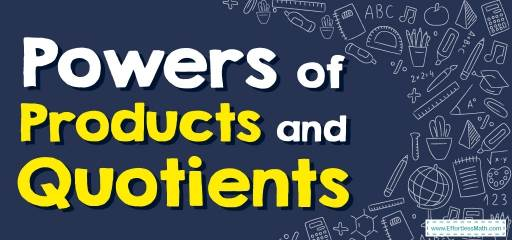# How to Solve Powers of Products and Quotients? (+FREE Worksheet!)

Learn how to take a product or a quotient to power by using powers of products and quotients rules.## Step by step guide to solve powers of products and quotients

• For any nonzero numbers $$a$$ and $$b$$ and any integer $$x$$,
$$\color{blue}{(ab)^x=a^x×b^x}$$.

The Absolute Best Book to Ace the College Algebra Course

### Powers of Products and Quotients – Example 1:

Simplify. $$(6x^2 y^4)^2=$$

Solution:

Use Exponent’s rules: $$\color{blue}{(ab)^x=a^x×b^x}$$
$$(6x^2y^4)^2=(6)^2(x^2)^2(y^4)^2=36x^{2×2}y^{4×2}=36x^4y^8$$

### Powers of Products and Quotients – Example 2:

Simplify. $$(\frac{5x}{2x^2})^2=$$

Solution:

First cancel the common factor: $$x→ (\frac{5x}{2x^2} )^2=(\frac{5}{2x})^2$$
Use Exponent’s rules: $$\color{blue}{(\frac{a}{b})^c=\frac{a^c}{b^c}}$$
Then: $$(\frac{5}{2x})^2=\frac{5^2}{(2x)^2} =\frac{25}{4x^2}$$

### Powers of Products and Quotients – Example 3:

Simplify. $$(3x^5 y^4)^2=$$

Solution:

Use Exponent’s rules: $$\color{ blue }{(x^a)^b=x^{a×b}}$$
$$(3x^5 y^4)^2=(3)^2 (x^5)^2 (y^4)^2=9x^{5×2} y^{4×2}=9x^{10} y^8$$

Best Algebra Prep Resource

### Powers of Products and Quotients – Example 4:

Simplify. $$(\frac{2x}{3x^2} )^2=$$

Solution:

First cancel the common factor: $$x→ (\frac{2x}{3x^2} )^2=(\frac{2}{3x})^2$$
Use Exponent’s rules: $$\color{blue}{(\frac{a}{b})^c=\frac{a^c}{b^c}}$$
Then: $$(\frac{2}{3x})^2=\frac{2^2}{(3x)^2 }=\frac{4}{9x^2 }$$

## Exercises for Solving Powers of Products and Quotients

### Simplify.

1. $$\color{blue}{(2x^6y^8)^2}$$
2. $$\color{blue}{(2x^3x)^3}$$
3. $$\color{blue}{(2x^9 x^6)^3}$$
4. $$\color{blue}{(5x^{10}y^3)^3}$$
5. $$\color{blue}{(4x^3 x^2)^2}$$
6. $$\color{blue}{(3x^3 5x)^2}$$

1. $$\color{blue}{4x^{12}y^{16}}$$
2. $$\color{blue}{8x^{12}}$$
3. $$\color{blue}{8x^{45} }$$
4. $$\color{blue}{125x^{30}y^9}$$
5. $$\color{blue}{16x^{10}}$$
6. $$\color{blue}{225x^8}$$

The Best Books to Ace Algebra

### What people say about "How to Solve Powers of Products and Quotients? (+FREE Worksheet!) - Effortless Math: We Help Students Learn to LOVE Mathematics"?

No one replied yet.

X
45% OFF

Limited time only!

Save Over 45%

SAVE $40 It was$89.99 now it is \$49.99